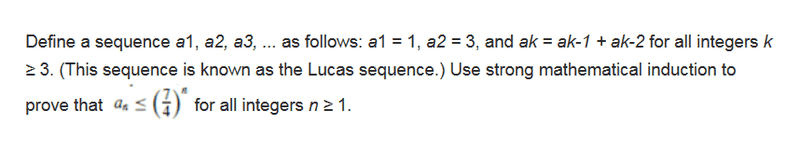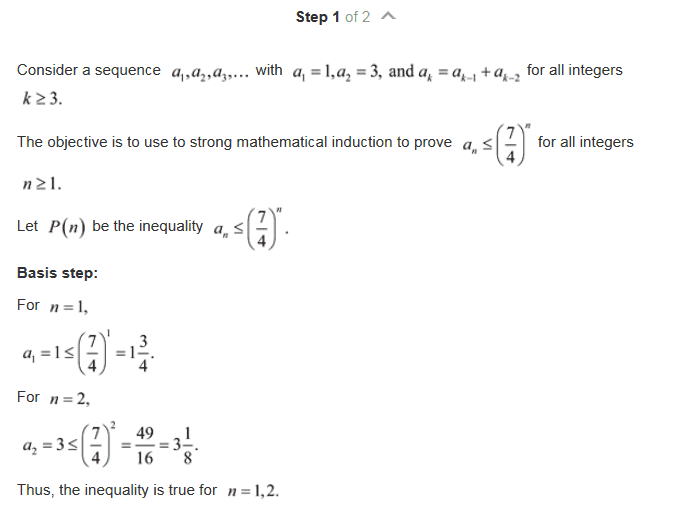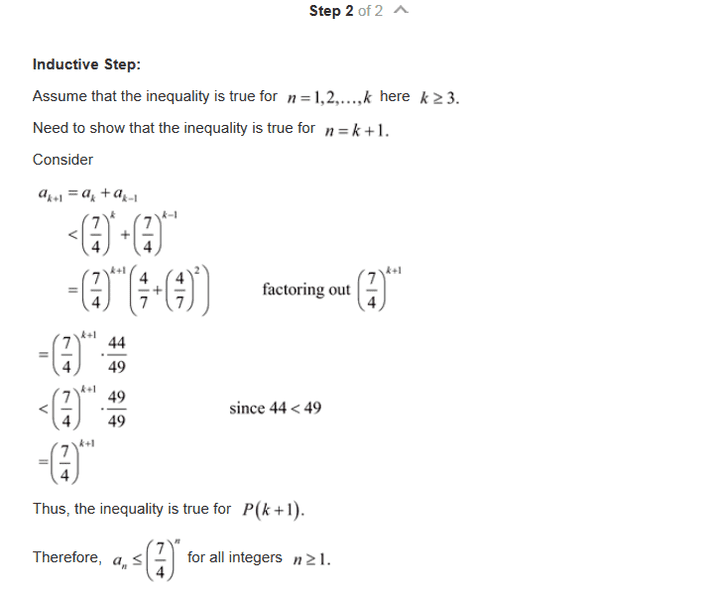# Discrete Math - Strong Induction Question

• Rosebud

## Homework Statement

Prove by Strong Mathematical Induction[/B]N/A

## The Attempt at a Solution

The steps to solving this problem are shown below. I understand all steps of the problem until the part where it says 44/49 becomes 49/49 since 44 < 49. Can someone please explain the logic behind that step? Thank you.Last edited:
If a>0 then a*(44/49) < a*(49/49).
Sort of like saying that f(x) = ax is strictly increasing.